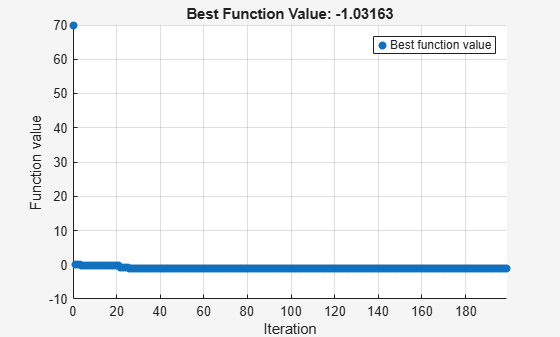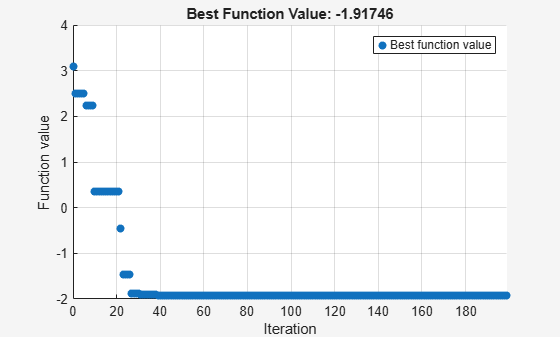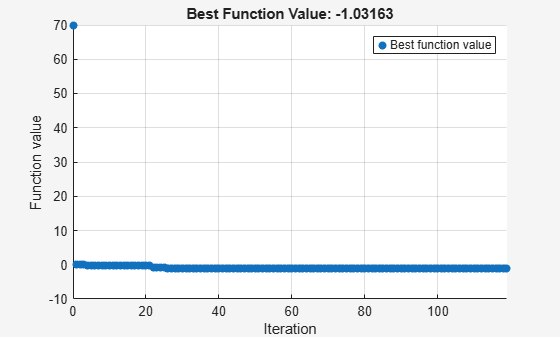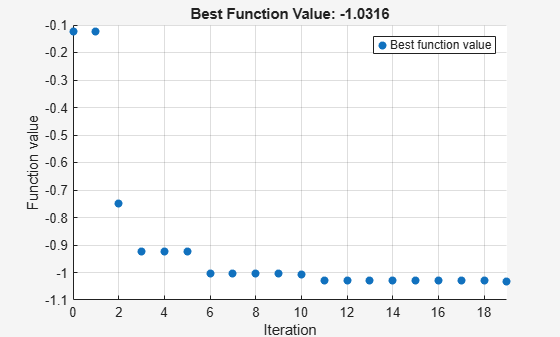# surrogateopt

Surrogate optimization for global minimization of time-consuming objective functions

## Syntax

``x = surrogateopt(objconstr,lb,ub)``
``x = surrogateopt(objconstr,lb,ub,intcon)``
``x = surrogateopt(___,options)``
``x = surrogateopt(problem)``
``x = surrogateopt(checkpointFile)``
``x = surrogateopt(checkpointFile,opts)``
``[x,fval] = surrogateopt(___)``
``[x,fval,exitflag,output] = surrogateopt(___)``
``[x,fval,exitflag,output,trials] = surrogateopt(___)``

## Description

The `surrogateopt` function is a global solver for time-consuming objective functions.

`surrogateopt` attempts to solve problems of the form

The solver searches for the global minimum of a real-valued objective function in multiple dimensions, subject to bounds, optional integer constraints, and optional nonlinear inequality constraints. `surrogateopt` is best suited to objective functions that take a long time to evaluate. The objective function can be nonsmooth. The solver requires finite bounds on all variables. The solver can optionally maintain a checkpoint file to enable recovery from crashes or partial execution, or optimization continuation after meeting a stopping condition.

example

````x = surrogateopt(objconstr,lb,ub)` searches for a global minimum of `objconstr(x)` in the region `lb <= x <= ub`. If `objconstr(x)` returns a structure, then `surrogateopt` searches for a minimum of `objconstr(x).Fval`, subject to `objconstr(x).Ineq <= 0`. NotePassing Extra Parameters (Optimization Toolbox) explains how to pass extra parameters to the objective function, if necessary. ```

example

````x = surrogateopt(objconstr,lb,ub,intcon)` requires that the variables listed in `intcon` take integer values. ```

example

````x = surrogateopt(___,options)` modifies the search procedure using the options in `options`. Specify `options` following any input argument combination in the previous syntaxes.```

example

````x = surrogateopt(problem)` searches for a minimum for `problem`, a structure described in `problem`.```

example

````x = surrogateopt(checkpointFile)` continues running the optimization from the state in a saved checkpoint file. See Work with Checkpoint Files.```

example

````x = surrogateopt(checkpointFile,opts)` continues running the optimization from the state in a saved checkpoint file, and replaces options in `checkpointFile` with those in `opts`. See Checkpoint File.```

example

````[x,fval] = surrogateopt(___)` also returns the best (smallest) value of the objective function found by the solver, using any of the input argument combinations in the previous syntaxes.```

example

````[x,fval,exitflag,output] = surrogateopt(___)` also returns `exitflag`, an integer describing the reason the solver stopped, and `output`, a structure describing the optimization procedure.```

example

````[x,fval,exitflag,output,trials] = surrogateopt(___)` also returns a structure containing all of the evaluated points and the objective function values at those points.```

## Examples

collapse all

Search for a minimum of the six-hump camel back function in the region `-2.1 <= x(i) <= 2.1`. This function has two global minima with the objective function value `-1.0316284...` and four local minima with higher objective function values.

```rng default % For reproducibility objconstr = @(x)(4*x(:,1).^2 - 2.1*x(:,1).^4 + x(:,1).^6/3 ... + x(:,1).*x(:,2) - 4*x(:,2).^2 + 4*x(:,2).^4); lb = [-2.1,-2.1]; ub = -lb; x = surrogateopt(objconstr,lb,ub)``````Surrogateopt stopped because it exceeded the function evaluation limit set by 'options.MaxFunctionEvaluations'. ```
```x = 1×2 0.0895 -0.7130 ```

Find the minimum of Rosenbrock's function

`$100\left(x\left(2\right)-x\left(1{\right)}^{2}{\right)}^{2}+\left(1-x\left(1\right){\right)}^{2}$`

subject to the nonlinear constraint that the solution lies in a disk of radius 1/3 around the point [1/3,1/3]:

$\left(x\left(1\right)-1/3{\right)}^{2}+\left(x\left(2\right)-1/3{\right)}^{2}\le \left(1/3{\right)}^{2}$.

To do so, write a function objconstr`(x)` that returns the value of Rosenbrock's function in a structure field `Fval`, and returns the nonlinear constraint value in the form $c\left(x\right)\le 0$ in the structure field `Ineq`.

`type objconstr`
```function f = objconstr(x) f.Fval = 100*(x(2) - x(1)^2)^2 + (1 - x(1))^2; f.Ineq = (x(1)-1/3)^2 + (x(2)-1/3)^2 - (1/3)^2; ```

Call `surrogateopt` using lower bounds of 0 and upper bounds of 2/3 on each component.

```lb = [0,0]; ub = [2/3,2/3]; [x,fval,exitflag] = surrogateopt(@objconstr,lb,ub)``````Surrogateopt stopped because it exceeded the function evaluation limit set by 'options.MaxFunctionEvaluations'. ```
```x = 1×2 0.6541 0.4277 ```
```fval = 0.1197 ```
```exitflag = 0 ```

Check the value of the nonlinear constraint at the solution.

`disp(objconstr(x).Ineq)`
``` 6.7195e-04 ```

The constraint function value is near zero, indicating that the constraint is active at the solution.

Find the minimum of the `ps_example` function for a two-dimensional variable `x` whose first component is restricted to integer values, and all components are between –5 and 5.

```intcon = 1; rng default % For reproducibility objconstr = @ps_example; lb = [-5,-5]; ub = [5,5]; x = surrogateopt(objconstr,lb,ub,intcon)``````Surrogateopt stopped because it exceeded the function evaluation limit set by 'options.MaxFunctionEvaluations'. ```
```x = 1×2 -5.0000 -0.0005 ```

Minimize the six-hump camel back function in the region `-2.1 <= x(i) <= 2.1`. This function has two global minima with the objective function value `-1.0316284...` and four local minima with higher objective function values.

To search the region systematically, use a regular grid of starting points. Set 120 as the maximum number of function evaluations. Use the `'surrogateoptplot'` plot function. To understand the `'surrogateoptplot'` plot, see Interpret surrogateoptplot.

```rng default % For reproducibility objconstr = @(x)(4*x(:,1).^2 - 2.1*x(:,1).^4 + x(:,1).^6/3 ... + x(:,1).*x(:,2) - 4*x(:,2).^2 + 4*x(:,2).^4); lb = [-2.1,-2.1]; ub = -lb; [Xpts,Ypts] = meshgrid(-3:3); startpts = [Xpts(:),Ypts(:)]; options = optimoptions('surrogateopt','PlotFcn','surrogateoptplot',... 'InitialPoints',startpts,'MaxFunctionEvaluations',120); x = surrogateopt(objconstr,lb,ub,options)``````Surrogateopt stopped because it exceeded the function evaluation limit set by 'options.MaxFunctionEvaluations'. ```
```x = 1×2 -0.0904 0.7127 ```

Create a problem structure representing the six-hump camel back function in the region `-2.1 <= x(i) <= 2.1`. Set 120 as the maximum number of function evaluations.

```rng default % For reproducibility objconstr = @(x)(4*x(:,1).^2 - 2.1*x(:,1).^4 + x(:,1).^6/3 ... + x(:,1).*x(:,2) - 4*x(:,2).^2 + 4*x(:,2).^4); options = optimoptions('surrogateopt','MaxFunctionEvaluations',120); problem = struct('objective',objconstr,... 'lb',[-2.1,-2.1],... 'ub',[2.1,2.1],... 'options',options,... 'solver','surrogateopt'); x = surrogateopt(problem)``````Surrogateopt stopped because it exceeded the function evaluation limit set by 'options.MaxFunctionEvaluations'. ```
```x = 1×2 0.0895 -0.7130 ```

Minimize the six-hump camel back function and return both the minimizing point and the objective function value. Set options to suppress all other display.

```rng default % For reproducibility objconstr = @(x)(4*x(:,1).^2 - 2.1*x(:,1).^4 + x(:,1).^6/3 ... + x(:,1).*x(:,2) - 4*x(:,2).^2 + 4*x(:,2).^4); lb = [-2.1,-2.1]; ub = -lb; options = optimoptions('surrogateopt','Display','off','PlotFcn',[]); [x,fval] = surrogateopt(objconstr,lb,ub,options)```
```x = 1×2 0.0895 -0.7130 ```
```fval = -1.0316 ```

Monitor the surrogate optimization process by requesting that `surrogateopt` return more outputs. Use the `'surrogateoptplot'` plot function. To understand the `'surrogateoptplot'` plot, see Interpret surrogateoptplot.

```rng default % For reproducibility objconstr = @(x)(4*x(:,1).^2 - 2.1*x(:,1).^4 + x(:,1).^6/3 ... + x(:,1).*x(:,2) - 4*x(:,2).^2 + 4*x(:,2).^4); lb = [-2.1,-2.1]; ub = -lb; options = optimoptions('surrogateopt','PlotFcn','surrogateoptplot'); [x,fval,exitflag,output] = surrogateopt(objconstr,lb,ub,options)``````Surrogateopt stopped because it exceeded the function evaluation limit set by 'options.MaxFunctionEvaluations'. ```
```x = 1×2 0.0895 -0.7130 ```
```fval = -1.0316 ```
```exitflag = 0 ```
```output = struct with fields: elapsedtime: 46.9309 funccount: 200 constrviolation: 0 ineq: [1x0 double] rngstate: [1x1 struct] message: 'Surrogateopt stopped because it exceeded the function evaluation limit set by ...' ```

Conclude a surrogate optimization quickly by setting a small maximum number of function evaluations. To prepare for the possibility of restarting the optimization, request all solver outputs.

```rng default % For reproducibility objconstr = @(x)(4*x(:,1).^2 - 2.1*x(:,1).^4 + x(:,1).^6/3 ... + x(:,1).*x(:,2) - 4*x(:,2).^2 + 4*x(:,2).^4); lb = [-2.1,-2.1]; ub = -lb; options = optimoptions('surrogateopt','MaxFunctionEvaluations',20); [x,fval,exitflag,output,trials] = surrogateopt(objconstr,lb,ub,options);``````Surrogateopt stopped because it exceeded the function evaluation limit set by 'options.MaxFunctionEvaluations'. ```

Optimize for another 20 function evaluations, starting from the previously evaluated points.

```options.InitialPoints = trials; [x,fval,exitflag,output,trials] = surrogateopt(objconstr,lb,ub,options);``````Surrogateopt stopped because it exceeded the function evaluation limit set by 'options.MaxFunctionEvaluations'. ```

By comparing the plots of these 40 function evaluations to those in Search for Global Minimum, you see that restarting surrogate optimization is not the same as having the solver run continuously.

To enable restarting surrogate optimization due to a crash or any other reason, set a checkpoint file name.

`opts = optimoptions('surrogateopt','CheckpointFile','checkfile.mat');`

Create an optimization problem and set a small number of function evaluations.

```rng default % For reproducibility objconstr = @(x)(4*x(:,1).^2 - 2.1*x(:,1).^4 + x(:,1).^6/3 ... + x(:,1).*x(:,2) - 4*x(:,2).^2 + 4*x(:,2).^4); lb = [-2.1,-2.1]; ub = -lb; opts.MaxFunctionEvaluations = 30; [x,fval,exitflag,output] = surrogateopt(objconstr,lb,ub,opts)``````Surrogateopt stopped because it exceeded the function evaluation limit set by 'options.MaxFunctionEvaluations'. ```
```x = 1×2 0.0067 -0.7343 ```
```fval = -0.9986 ```
```exitflag = 0 ```
```output = struct with fields: elapsedtime: 11.7528 funccount: 30 constrviolation: 0 ineq: [1×0 double] rngstate: [1×1 struct] message: 'Surrogateopt stopped because it exceeded the function evaluation limit set by ↵'options.MaxFunctionEvaluations'.' ```

Set options to use 100 function evaluations (which means 70 more than already done) and restart the optimization.

```opts.MaxFunctionEvaluations = 100; [x2,fval2,exitflag2,output2] = surrogateopt('checkfile.mat',opts)``````Surrogateopt stopped because it exceeded the function evaluation limit set by 'options.MaxFunctionEvaluations'. ```
```x2 = 1×2 0.0895 -0.7130 ```
```fval2 = -1.0316 ```
```exitflag2 = 0 ```
```output2 = struct with fields: elapsedtime: 64.8767 funccount: 100 constrviolation: 0 ineq: [1×0 double] rngstate: [1×1 struct] message: 'Surrogateopt stopped because it exceeded the function evaluation limit set by ↵'options.MaxFunctionEvaluations'.' ```

## Input Arguments

collapse all

Objective function and nonlinear constraint, specified as a function handle or function name. `objconstr` accepts a single argument `x`, where `x` is a row vector. `objconstr` returns one of the following:

• Real scalar `fval = objconstr(x)`.

• Structure. If the structure contains the field `Fval`, then `surrogateopt` attempts to minimize `objconstr(x).Fval`. If the structure contains the field `Ineq`, then `surrogateopt` attempts to make all components of that field nonpositive: ```objconstr(x).Ineq <= 0``` for all entries. `objconstr(x)` must include either the `Fval` or `Ineq` fields, or both. `surrogateopt` ignores other fields.

For examples using a nonlinear constraint, see Solve Problem with Nonlinear Constraints, Surrogate Optimization with Nonlinear Constraint, and Solve Feasibility Problem. For information on converting between the `surrogateopt` structure syntax and other solvers, see `packfcn` and Convert Nonlinear Constraints Between surrogateopt Form and Other Solver Forms.

Data Types: `function_handle` | `char` | `string`

Lower bounds, specified as a finite real vector. `lb` represents the lower bounds element-wise in `lb `` x `` ub`. The lengths of `lb` and `ub` must be equal to the number of variables that `objconstr` accepts.

### Caution

Although `lb` is optional for most solvers, `lb` is a required input for `surrogateopt`.

### Note

`surrogateopt` allows equal entries in `lb` and `ub`. For each `i` in `intcon`, you must have `ceil(lb(i)) <= floor(ub(i))`. See Construct Surrogate Details.

Example: `lb = [0;-20;4]` means ```x(1) ≥ 0```, `x(2) ≥ -20`, ```x(3) ≥ 4```.

Data Types: `double`

Upper bounds, specified as a finite real vector. `ub` represents the upper bounds element-wise in `lb `` x `` ub`. The lengths of `lb` and `ub` must be equal to the number of variables that `objconstr` accepts.

### Caution

Although `ub` is optional for most solvers, `ub` is a required input for `surrogateopt`.

### Note

`surrogateopt` allows equal entries in `lb` and `ub`. For each `i` in `intcon`, you must have `ceil(lb(i)) <= floor(ub(i))`. See Construct Surrogate Details.

Example: `ub = [10;-20;4]` means ```x(1) ≤ 10```, `x(2) ≤ -20`, ```x(3) ≤ 4```.

Data Types: `double`

Integer variables, specified as a vector of positive integers with values from `1` to the number of problem variables. Each value in `intcon` represents an `x` component that is integer-valued.

Example: To specify that the even entries in `x` are integer-valued, set `intcon` to `2:2:nvars`.

Data Types: `double`

Options, specified as the output of `optimoptions`.

OptionDescriptionValues
`CheckpointFile`

File name for checkpointing and restarting optimization. The file has the `.mat` data type. See Work with Checkpoint Files.

Checkpointing takes time. This overhead is especially noticeable for functions that otherwise take little time to evaluate.

File name or file path, given as a string or character array. If you specify a file name without a path, `surrogateopt` saves the checkpoint file in the current folder.

`ConstraintTolerance`Tolerance on nonlinear constraints, measured as the maximum of all nonlinear constraint function values, where positive values indicate a violation. This tolerance is an absolute (not relative) tolerance; see Tolerances and Stopping Criteria (Optimization Toolbox).Positive scalar. Default is `1e-3`.
`Display`

Level of display returned at the command line.

• `'final'` (default) — Exit message at the end of the iterations.

• `'off'` or the equivalent `'none'` — No display.

• `'iter'` — Iterative display; see Command-Line Display.

`InitialPoints`Initial points for solver.

Matrix of initial points, where each row is one point. Or, a structure with field `X`, whose value is a matrix of initial points, and these optional fields:

• `Fval`, a vector containing the values of the objective function at the initial points

• `Ineq`, a matrix containing nonlinear inequality constraint values

See Algorithm Control. Default is `[]`.

`MaxFunctionEvaluations`Maximum number of objective function evaluations, a stopping criterion.Positive integer. Default is `max(200,50*nvar)`, where `nvar` is the number of problem variables.
`MaxTime`Maximum running time in seconds. The actual running time can exceed `MaxTime` because of the time required to evaluate an objective function or because of parallel processing delays.Positive scalar. Default is `Inf`.
`MinSampleDistance`Minimum distance between trial points generated by the adaptive sampler. See Surrogate Optimization Algorithm.Positive scalar. Default is `1e-3`.
`MinSurrogatePoints`Minimum number of random sample points to create at the start of a surrogate creation phase. See Surrogate Optimization Algorithm.Integer at least `nvar` + 1. Default is `max(20,2*nvar)`, where `nvar` is the number of problem variables.
`ObjectiveLimit`Tolerance on the objective function value. If a calculated objective function value is less than `ObjectiveLimit`, the algorithm stops.Double scalar value. Default is `-Inf`.
`OutputFcn`Output function to report on solver progress or to stop the solver. See Output Function.Function name, function handle, or cell array of function names or handles. Default is `[]`.
`PlotFcn`

Plot function to display solver progress or to stop solver. See Plot Function.

Function name, function handle, or cell array of function names or handles. Built-in plot functions are:

• `'optimplotfvalconstr'` (default) — Plot the best feasible objective function value found as a line plot. If there is no objective function, plot the maximum nonlinear constraint violation as a line plot.

• The plot shows infeasible points as red and feasible points as blue.

• If there is no objective function, the plot title shows the number of feasible solutions.

• `'optimplotfval'` — Plot the best objective function value found as a line plot.

• `'optimplotx'` — Plot the best solution found as a bar chart.

• `'surrogateoptplot'` — Plot the objective function value at each iteration, showing which phase of the algorithm produces the value and the best value found both in this phase and overall. See Interpret surrogateoptplot.

`UseParallel`Boolean value indicating whether to compute objective function values in parallel.Boolean. Default is `false`. For algorithmic details, see Parallel surrogateopt Algorithm.

Example: ```options = optimoptions('surrogateopt','Display','iter','UseParallel',true)```

Problem structure, specified as a structure with these fields:

• `objective` — Objective function, which can include nonlinear constraints, specified as a function name or function handle

• `lb` — Lower bounds for `x`

• `ub` — Upper bounds for `x`

• `solver``'surrogateopt'`

• `options` — Options created with `optimoptions`

• `rngstate` — Optional field to reset the state of the random number generator

• `intcon` — Optional field specifying integer-valued `x` components

### Note

All fields in `problem` are required except `rngstate` and `intcon`.

Data Types: `struct`

Path to a checkpoint file, specified as a string or character vector. A checkpoint file has the `.mat` extension. If you specify a file name without a path, `surrogateopt` uses a checkpoint file in the current folder.

A checkpoint file stores the state of an optimization for resuming the optimization. `surrogateopt` updates the checkpoint file at each function evaluation, so you can resume the optimization even when `surrogateopt` halts prematurely. For an example, see Restart Surrogate Optimization from Checkpoint File.

`surrogateopt` creates a checkpoint file when it has a valid `CheckpointFile` option.

You can change some options when resuming from a checkpoint file. See `opts`.

The data in a checkpoint file is in `.mat` format. To avoid errors or other unexpected results, do not modify the data before calling `surrogateopt`.

### Warning

Do not resume `surrogateopt` from a checkpoint file created with a different MATLAB® version. `surrogateopt` can throw an error or give inconsistent results.

Example: `'checkfile.mat'`

Example: ```"C:\Program Files\MATLAB\docs\checkpointNov2019.mat"```

Data Types: `char` | `string`

Options for resuming optimization from the checkpoint file, specified as `optimoptions` options (from a restricted set) that you can change from the original options. The options you can change are:

• `CheckpointFile`

• `Display`

• `MaxFunctionEvaluations`

• `MaxTime`

• `MinSurrogatePoints`

• `ObjectiveLimit`

• `OutputFcn`

• `PlotFcn`

• `UseParallel`

Example: ```opts = optimoptions(options,'MaxFunctionEvaluations',400);```

## Output Arguments

collapse all

Solution, returned as a real vector. `x` has the same length as `lb` and `ub`.

Objective function value at the solution, returned as a real number.

• When `objconstr` returns a scalar, `fval` is the scalar `objconstr(x)`.

• When `objconstr` returns a structure, `fval` is the value `objconstr(x).Fval`, the objective function value at `x` (if this value exists).

Reason `surrogateopt` stopped, returned as one of the integer values described in this table.

Exit FlagDescription

`10`

All upper bounds `ub` are equal to the lower bounds `lb`. `surrogateopt` returns the feasible point without performing any optimization.

`1`

The objective function value is less than `options.ObjectiveLimit`. This exit flag takes precedence over exit flag `10` when both apply.

`0`

The number of function evaluations exceeds `options.MaxFunctionEvaluations` or the elapsed time exceeds `options.MaxTime`. If the problem has nonlinear inequalities, the solution is feasible.

`-1`

The optimization is terminated by an output function or plot function.

`-2`

No feasible point is found due to one of the following:

• A lower bound `lb(i)` exceeds a corresponding upper bound `ub(i)`. In this case, `surrogateopt` returns `x = []` and ```fval = []```.

• `lb = ub` and the point `lb` is infeasible. In this case, `x = lb`, and ```fval = objconstr(x).Fval```.

• `lb <= ub` and no feasible solution is found. In this case, `x` is the point of least infeasibility, and ```fval = objconstr(x).Fval```.

Information about the optimization process, returned as a structure with these fields:

• `funccount` — Total number of function evaluations.

• `elapsedtime` — Time spent running the solver in seconds, as measured by `tic`/`toc`.

• `message` — Reason why the algorithm stopped.

• `constrviolation` — Maximum constraint violation, if any. ```constrviolation = max(output.ineq)```.

• `ineq` — Inequality constraint value at the solution `x`. If `objconstr` returns a structure, then `ineq` = `objconstr(x).Ineq`. Otherwise, `ineq` is empty.

• `rngstate` — State of the MATLAB random number generator just before the algorithm starts. Use this field to reproduce your results. See Reproduce Results, which discusses using `rngstate` for `ga`.

Points evaluated, returned as a structure with these fields:

• `X` — Matrix with `nvars` columns, where `nvars` is the length of `lb` or `ub`. Each row of `X` represents one point evaluated by `surrogateopt`.

• `Fval` — Column vector, where each entry is the objective function value of the corresponding row of `X`.

• `Ineq` — Matrix with each row representing the constraint function values of the corresponding row of `X`.

The `trials` structure has the same form as the `options.InitialPoints` structure. So, you can continue an optimization by passing the `trials` structure as the `InitialPoints` option.

## Algorithms

`surrogateopt` repeatedly performs these steps:

1. Create a set of trial points by sampling `MinSurrogatePoints` random points within the bounds, and evaluate the objective function at the trial points.

2. Create a surrogate model of the objective function by interpolating a radial basis function through all of the random trial points.

3. Create a merit function that gives some weight to the surrogate and some weight to the distance from the trial points. Locate a small value of the merit function by randomly sampling the merit function in a region around the incumbent point (best point found since the last surrogate reset). Use this point, called the adaptive point, as a new trial point.

4. Evaluate the objective at the adaptive point, and update the surrogate based on this point and its value. Count a "success" if the objective function value is sufficiently lower than the previous best (lowest) value observed, and count a "failure" otherwise.

5. Update the dispersion of the sample distribution upwards if three successes occur before `max(nvar,5)` failures, where `nvar` is the number of dimensions. Update the dispersion downwards if `max(nvar,5)` failures occur before three successes.

6. Continue from step 3 until all trial points are within `MinSampleDistance` of the evaluated points. At that time, reset the surrogate by discarding all adaptive points from the surrogate, reset the scale, and go back to step 1 to create `MinSurrogatePoints` new random trial points for evaluation.

For details, see Surrogate Optimization Algorithm.